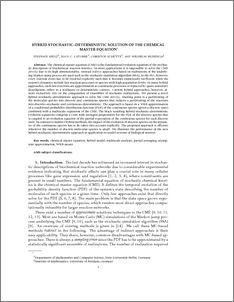Repository: Freie Universität Berlin, Math Department

# Hybrid Stochastic–Deterministic Solution of the Chemical Master Equation

Menz, S. and Latorre, J.C. and Schütte, Ch. and Huisinga, W. (2012) Hybrid Stochastic–Deterministic Solution of the Chemical Master Equation. SIAM Interdisciplinary Journal Multiscale Modeling and Simulation (MMS), 10 (4). pp. 1232-1262.Preview
PDF - Submitted Version
983kB

Official URL: http://dx.doi.org/10.1137/110825716

## Abstract

The chemical master equation (CME) is the fundamental evolution equation of the stochastic description of biochemical reaction kinetics. In most applications it is impossible to solve the CME directly due to its high dimensionality. Instead indirect approaches based on realizations of the underlying Markov jump process are used such as the stochastic simulation algorithm (SSA). In the SSA, however, every reaction event has to be resolved explicitly such that it becomes numerically inefficient when the system’s dynamics include fast reaction processes or species with high population levels. In many hybrid approaches, such fast reactions are approximated as continuous processes or replaced by quasi-stationary distributions either in a stochastic or deterministic context. Current hybrid approaches, however, almost exclusively rely on the computation of ensembles of stochastic realizations. We present a novel hybrid stochastic–deterministic approach to solve the CME directly. Starting point is a partitioning of the molecular species into discrete and continuous species that induces a partitioning of the reactions into discrete–stochastic and continuous–deterministic. The approach is based on a WKB approximation of a conditional probability distribution function (PDF) of the continuous species (given a discrete state) combined with a multiscale expansion of the CME. The black resulting hybrid stochastic–deterministic evolution equations comprise a CME with averaged propensities for the PDF of the discrete species that is coupled to an evolution equation of the partial expectation of the continuous species for each discrete state. In contrast to indirect hybrid methods, the impact of the evolution of discrete species on the dynamics of the continuous species has to be taken into account explicitly. The proposed approach is efficient whenever the number of discrete molecular species is small. We illustrate the performance of the new hybrid stochastic–deterministic approach in application to model systems of biological interest.

Item Type: Article Physical Sciences > Chemistry > Physical ChemistryMathematical and Computer Sciences > Mathematics > Engineering/Industrial Mathematics Department of Mathematics and Computer Science > Institute of MathematicsDepartment of Mathematics and Computer Science > Institute of Mathematics > Cellular Mechanics GroupDepartment of Mathematics and Computer Science > Institute of Mathematics > BioComputing Group 1039 BioComp Admin 24 Feb 2011 11:27 03 Mar 2017 14:41

Repository Staff Only: item control page